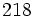# General linear group:GL(2,5)

View a complete list of particular groups (this is a very huge list!)[SHOW MORE]

## Definition

The group, denoted$GL(2,5)$ or$GL_2(5)$, is defined as the general linear group of degree two over the field of five elements.

## GAP implementation

### Group ID

This group has ID$218$ among the groups of order$480$. It can be defined using GAP's SmallGroup function:

`SmallGroup(480,218)`

### Other definitions

The group can also be defined using GAP's GeneralLinearGroup function:

`GL(2,5)`